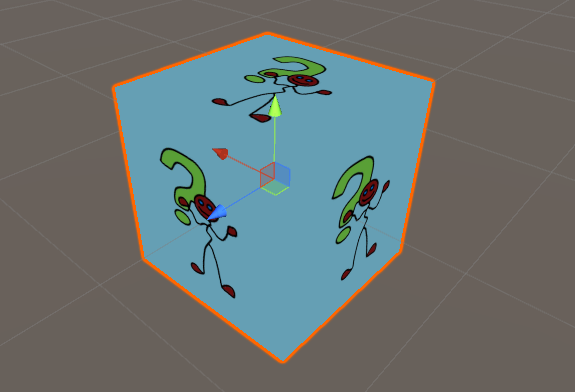## Summary

In the last three tutorials I explained some of the cornerstones of how shaders work. In this one I show you how to fill in the rest.

The main thing I didn’t show was actual executed code. Thats because you don’t need much code to get a shader running at first and all of the fancy code is in specialized tutorials.## What we have so far

Everything here should be explained in one of the previous three tutorials. Feel free to tell me if you think otherwise.

``````Shader "Tutorial/001-004_Basic_Unlit"{
//show values to edit in inspector
Properties{
_Color ("Tint", Color) = (0, 0, 0, 1)
_MainTex ("Texture", 2D) = "white" {}
}

//the material is completely non-transparent and is rendered at the same time as the other opaque geometry
Tags{ "RenderType"="Opaque" "Queue"="Geometry" }

Pass{
CGPROGRAM

//texture and transforms of the texture
sampler2D _MainTex;
float4 _MainTex_ST;

//tint of the texture
fixed4 _Color;

struct appdata{
float4 vertex : POSITION;
float2 uv : TEXCOORD0;
};

//the data thats passed from the vertex to the fragment shader and interpolated by the rasterizer
struct v2f{
float4 position : SV_POSITION;
float2 uv : TEXCOORD0;
};

ENDCG
}
}
Fallback "VertexLit"
}
``````

## Setting up the shader stages

Each of the shader stages is represented as a hlsl function. To define which function in your program represent the stages you add `#pragma` statements too your program. Important for the vertex stage is that it takes in the vertex data and returns the interpolators and important for the fragment stage is that it takes in the interpolators and returns a vector which writes into the render target. So this looks something like this:

``````//define vertex and fragment shader functions
#pragma vertex vert
#pragma fragment frag

v2f vert(appdata v){
//vertex stage stuff
}

fixed4 frag(v2f i) : SV_TARGET{
//fragment stage code
}
``````

## Vertex stage

At the start of the function that defines our vertex stage we create the interpolator struct we’ll return at the end. Then we’ll fill it with data and return it.

The main job of the vertex stage is to transform the vertex positions from local objectspace to clipspace where they can be rendered. This is done internally via a matrix multiplication, but we don’t have to understand that yet since unity gives us functions do the matrix multiplication for us. To use those macros (and lots of other useful code) in our shader we import a include file via `#include "UnityCG.cginc"`. The function to transform a position from local to clip space is called `UnityObjectToClipPos`. The UnityCG file also has a macro (works similar to functions) that helps us transform the uv coordinates that are passed with the vertex data into uv coordinates that respect the tiling and offset that we set in the editor, it is named `TRANSFORM_TEX` and takes the base uv coordinates as well as the name of the texture as arguments.

With that code applied the vertex function should look something like this:

``````//the vertex shader function
v2f vert(appdata v){
v2f o;
//convert the vertex positions from object space to clip space so they can be rendered correctly
o.position = UnityObjectToClipPos(v.vertex);
//apply the texture transforms to the UV coordinates and pass them to the v2f struct
o.uv = TRANSFORM_TEX(v.uv, _MainTex);
return o;
}
``````

## Fragment stage

In the fragment stage we take in the interpolators and use them as well as uniform variables to figure out what color that pixel should have. The simplest version of that could just be `return float4(1,1,1,1);` for a completely white mesh, but in most cases we want to use a bit more complicated result and use textures.

To access textures we usually use the `tex2D` function which takes in the texture as its first argument and the uv coordinates as the second argument and then returns the color at that coordinate. In this example we’ll save the resulting color, multiply it with a color we defined as a uniform variable and return the result.

``````//the fragment shader function
fixed4 frag(v2f i) : SV_TARGET{
//read the texture color at the uv coordinate
fixed4 col = tex2D(_MainTex, i.uv);
//multiply the texture color and tint color
col *= _Color;
//return the final color to be drawn on screen
return col;
}
``````

## Source

``````Shader "Tutorial/001-004_Basic_Unlit"{
//show values to edit in inspector
Properties{
_Color ("Tint", Color) = (0, 0, 0, 1)
_MainTex ("Texture", 2D) = "white" {}
}

//the material is completely non-transparent and is rendered at the same time as the other opaque geometry
Tags{ "RenderType"="Opaque" "Queue"="Geometry" }

Pass{
CGPROGRAM

#include "UnityCG.cginc"

//define vertex and fragment shader functions
#pragma vertex vert
#pragma fragment frag

//texture and transforms of the texture
sampler2D _MainTex;
float4 _MainTex_ST;

//tint of the texture
fixed4 _Color;

struct appdata{
float4 vertex : POSITION;
float2 uv : TEXCOORD0;
};

//the data thats passed from the vertex to the fragment shader and interpolated by the rasterizer
struct v2f{
float4 position : SV_POSITION;
float2 uv : TEXCOORD0;
};

v2f vert(appdata v){
v2f o;
//convert the vertex positions from object space to clip space so they can be rendered correctly
o.position = UnityObjectToClipPos(v.vertex);
//apply the texture transforms to the UV coordinates and pass them to the v2f struct
o.uv = TRANSFORM_TEX(v.uv, _MainTex);
return o;
}

fixed4 frag(v2f i) : SV_TARGET{
//read the texture color at the uv coordinate
fixed4 col = tex2D(_MainTex, i.uv);
//multiply the texture color and tint color
col *= _Color;
//return the final color to be drawn on screen
return col;
}

ENDCG
}
}
Fallback "VertexLit"
}
``````

You can also find me on twitter at @totallyRonja. If you liked my tutorial and want to support me you can do that on Patreon (patreon.com/RonjaTutorials) or Ko-Fi (ko-fi.com/RonjaTutorials).